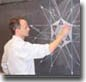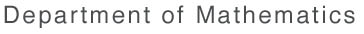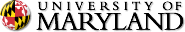This page is not longer being maintained. Please visit the new UMD Mathematics website at www-math.umd.edu.Math Home > Research > Seminars > Numerical Analysis Seminar > [ Search | Contact | Help! ]

# NUMERICAL ANALYSIS SEMINAR

### Department of Mathematics, University of Maryland, College Park

```                 F A L L   1 9 9 3   S C H E D U L E

-----------------------------------------------------------------
Sept. 16:  Mechanics of DNA supercoiling
-----------------------------------------------------------------
Dr. Yang Yang, Naval Research Lab.

DNA usually occurs as a double-helix with two complementary nucleotide
chains winding around a common axis. In cells, the common axis of the
DNA often folds into a supercoiled form that is linked to its
biological activities. The theories of mechanics which haven been
widely used in design of buildings, bridges and airplanes may provide
important quantitative analysis for these biological processes.
In the present approach a long DNA is modeled as a thin elastic rod.
Even for such a relatively simple model we still face a great deal
of difficulty in overcoming non-linearity and self-contact resulting
from DNA supercoiling. A finite element scheme based on Kirchhoff's
rod theory is newly formulated to address these problems. Until now
most of these problems have not been fully understood. There is no
shortage of interesting mathematics and physics problems here.

-----------------------------------------------------------------
Sept. 23: Amending the AR Method for Frequency Detection
by a Fixed Point Idea
-----------------------------------------------------------------
Prof. Benjamin Kedem,
Dept. of Math. and Systems Research Center, UMCP

It is well known that the AR method for multiple frequency
estimation known as Prony's method, gives asymptotically biased
estimates.  In an attempt to improve on this problem,
Dragosevic and Stankovic (1989) couple the AR method
with an iterative scheme, discussed by Kay (1984), and an
all-pole filter with poles inside the unit circle.
But the asymptotic bias still persists.

It is shown, however,
that if the least squares estimator of the AR parameters
is parametrized by using a parametric filter which possesses a
certain ``fundamental property", a consistent estimator is
obtained from the fixed point of the parametrized least squares
(random) estimator.

In particular, the all-pole filter considered by
Dragosevic and Stankovic can be reparametrized so
that it too possesses the ``fundamental property" and thus leads to
consistent estimates. Experimental results with the reparametrized
all-pole filter show that the modified method has high resolution,
and that its overall performance is quite remarkable.

------------------------------------------------------------------------
Sept. 30: Extrapolation Quadrature over Triangles
------------------------------------------------------------------------
Prof. J. N. Lyness, Argonne National Laboratory

In this talk, I shall treat parts of the theory of extrapolation
quadrature. First I shall provide a brief one-dimensional background.
Then I shall treat numerical quadrature over triangles. In each case
I shall treat first the theory relating to regular integrands and
then the corresponding theory for singular integrands with emphasis on
the "full corner singularity". Some recent results relating to
integration over curved surfaces will be mentioned.

------------------------------------------------------------------------
Oct. 7: Compilers and Runtime Support for Unstructured Scientific Problems
------------------------------------------------------------------------
Prof. J. Saltz, Dept. of Computer Science, UMCP

We are developing methods that make it possible to produce portable compilers
targeted at scalable architectures that generate efficient code for sparse,
irregular, adaptive and block structured computations.

The development of sophisticated runtime support forms the foundation of our
research effort.  We have developed the CHAOS library, a runtime support
library that couples partitioners to compilers, partitions loop iterations,
remaps data and index arrays, and generates optimized communication schedules.
We have implemented address translation methods which support irregular
mappings by using highly optimized page-based translation tables.  We have also
developed a partitioner library, (PIECES library) containing a wide range of
efficient partitioners which are based on  data access patterns of a problem,
computational load information and problem geometry.

Using the CHAOS library and the Syracuse Fortran 90D compiler we have developed
a prototype distributed memory compiler able to generate efficient code for
templates extracted from adaptive problems.  One particularly innovative
feature associated with this compiler is our simple but robust method of
verifying that the communication preprocessing associated with a given data
access pattern can be reused.

This compiler also generates code for problems with irregularly coupled regular
meshes. We have used this compiler to parallelize a kernel abstracted from a
block structured Navier-Stokes solver and a full semi-coarsening multigrid
code.  We have also applied CHAOS directly to parallelize full adaptive
applications codes.  A large scale test of our methods involved porting a 120K
line molecular dynamics code, CHARMM, onto the Intel iPSC/860. We obtained
results that were better than any other known parallel implementation of
CHARMM.

------------------------------------------------------------------------
Oct. 14:  Successive Band Reduction and Tridiagonalization
------------------------------------------------------------------------
Dr. Xiaobai Sun, Argonne National Laboratory

Reduction to tridiagonal form is a major step in many algorithms
for symmetric eigenvalue problems. The conventional methods for
tridigonalization eliminate subdiagonals either one at a time or
all at a time. We have developed a new approach that, by eliminating
several (but not necessarily all) subdiagonals at a time, leads to
algorithms with lower computational and storage complexity and
increased scope for parallelism. This class of algorithms also naturally
lends itself to the use of block orthogonal transformations which
decrease the overall communication complexity and exploit the
architectural features of high-performance superscalar processors.
In addition, the successive band reduction algorithm can also be very
advantageously used to compute the invariant subspaces of matrices arising
in the so-called invariant subspace decomposition algorithm, where the
eigenvalue problem is repeatedly decoupled by computed invariant subspaces.
We will present computational results on Delta, SP-1, and Paragon,
where we implemented our successive band reduction algorithm using a
two-dimensional block torus wrap mapping.

------------------------------------------------------------------------
Oct. 21: Numerical Solution of 4th Order Sturm-Liouville Problems
------------------------------------------------------------------------
Prof. M. Marletta, University of Leicester, England

The most successful methods for solving second order Sturm-Liouville
Problems rely on the well-developed Sturm Oscillation Theory for these
problems. In this talk I will present some oscillation results which
may be used as the basis of solution methods for 4th order
Sturm-Liouville eigenvalue problems. Some of these are a consequence
of the Hamiltonian structures underlying the problems; others are
unique to fourth order problems. This is joint work with Leon Greenberg.

------------------------------------------------------------------------
Oct. 28: Global Search and Continuation in Structural Elasticity
------------------------------------------------------------------------
Dr. Gabor Domokos, Technical University of Budapest, Hungary

The state-change of elastic bar structures subject to slowly varying
load is parametrized by physical time, however, since the speed of load
variation is not specified, physical time is not a good choice for the
independent variable. There is a "quasi-time" in statics which is quite
different from physical time.  The first goal of this talk is to define
this quasi-time mathematically for elastic bar structures.
As a tool for this definition we introduce the phase space of the
boundary value problem (BVP), being analogous to the phase space of the
initial value problem (IVP). The investigation of the principal
similarities and differences between the IVP and the BVP phase space
lead us to the construction of global search and continuation
algorithms which neither rely on iteration techniques, nor do they
accumulate errors.
We will demonstrate the global behaviour of some sample problems by
applying the aforementioned numerical methods.

------------------------------------------------------------------------
Nov. 4: Parallel Function Decomposition and Space Decomposition Method
------------------------------------------------------------------------
Dr. Xue-Cheng Tai, Univ. of Jyvaskyla, Finland

Some methods which we call function decomposition methods and space
decomposition methods are developed. These methods deal with a convex
programming problem, i.e., a minimization problem of a convex function
over a space or a convex set.  If the function can be decomposed into
the sum of subspaces, then parallel methods can be used for the
minimization. The intention of this research is to give a general
convergence theory for domain decomposition methods and to show a
relationship between the domain decomposition method and the
traditional splitting method. Especially, we intend to use this theory
to extend domain decomposition methods for some nonlinear problems.

To use the developed algorithms for practical problems, there are
different ways to decompose a function and to decompose a space. Many
partial differential equations can be formulated as a minimization
problem in some way. Therefore we get some parallel methods for partial
differential equations.  The method is not restricted to linear
problems, nonlinear problems are naturally included in the theory.
Applications to linear and quasilinear self-adjoint elliptic equations,
to strongly nonlinear elliptic equations like the p-Laplace equation,
to the Stokes equation, and to variational problems like the obstacle
problem are discussed. With the applications we show that the the
classical alternating direction method and local one-dimensional method
are one way to decompose a function. The domain decomposition methods
are ways to decompose a function and also ways to decompose a space.
One observation is that the Schwarz alternating method for the
overlapping domain decomposition is a Gauss-Seidel method. If we
replace the Gauss-Seidel method by the Jacobi method we will get
parallel domain decomposition methods. Convergence of the Jacobi method
for general space decomposition is proved here.

------------------------------------------------------------------------
Nov.11: Finite Element Solution to the Exteriour Helmholtz Problem:
A One Dimensional Study of the h- and h-p-Versions
------------------------------------------------------------------------
Dr. Frank Ihlenburg, Institute for Physical Science and Technology, UMCP

While proofs of stability and convergence for the FE-solution of the
exteriour Helmholtz problem have been given previously, there exists a
certain gap between the asymptotic theorems of numerical analysis and
"rules of the thumb" that are applied by engineers to choose the
meshwidth in practical computations.  On the one hand, the assumptions
of the analytical results do not lead to practically applicable rules
if the wavenumber is high. On the other hand, the rules used so far in
applications of the h-version are reported to fail in the very case of
high wavenumber. The talk will
* present analytical results for the weak formulation of the Helmholtz problem
* investigate by numerical experimentation if the assumptions of the theorems
are due to technicalities or inherent to the problem
* draw practical conclusions for the application of the h- and h-p-version in
the case of high wavenumbers.

------------------------------------------------------------------------
Nov.18: Scientific Libraries on Scalable Architectures
------------------------------------------------------------------------
Dr. Lennart Johnsson, Thinking Machines Corporation

Maximizing locality of reference and minimizing network contention while
maintaining load balance is key to efficient computations on massively
parallel processors.  The memory hierarchy in each node includes registers,
cache, DRAM pages, and the maintenance of memory maps if not all of memory
is mapped at any given time.  In addition there is remote memory with varying
access characteristics.  The efficient management of memory hierarchies of
this type pose a serious challange, often beyond state-of-the-art compilers
and run-time systems.  Thus, memory management has become a significant
portion of scientific libraries for distributed memory architectures.  Tools
for data (re)distribution is an important component for good performance on
unstructured grid problems.

Libraries for distributed memory archietctures must accept data structures
distributed in arbitrary ways in memory and must exploit concurrency in
computations on single instances of objects as well as the concurrency offered
by computations on multiple instances.  The machine size and configuration
must be transparent to the programmer.  We will discuss how these challenges
have been addressed in the Connection Machine Scientific Software Library
(CMSSL).

------------------------------------------------------------------------
Dec. 2: Inexact and Preconditioned Uzawa Algorithms for Saddle Point Problems
------------------------------------------------------------------------
Prof. Howard Elman, Dept. of Computer Science, UMCP

We develop and analyze variants of the Uzawa algorithm for solving symmetric
indefinite linear systems of the type arising from discretization of the
Stokes equations.  Each step of this algorithm requires the solution of a
symmetric positive-definite system of linear equations.  We prove that if this
computation is replaced by an approximate solution produced by an arbitrary
iterative method, then with relatively modest requirements on the accuracy of
the approximate solution, the resulting inexact Uzawa algorithm is convergent
with convergence rate close to that of the exact algorithm.  In addition, for
mixed finite element discretizations of the Stokes equations, we show how to
precondition the system using a submatrix of the pressure mass matrix so that
convergence of both the exact and inexact Uzawa algorithms is improved.  The
analysis is supplemented by numerical results demonstrating the effectiveness
of the inexact and preconditioned algorithms.

-----------------------------------------------------------------------------
Dec. 9: Wavelet Bases for Boundary Element Methods
-----------------------------------------------------------------------------
Prof. Tobias von Petersdorff, Department of Mathmematics, UMCP

Boundary Element Methods lead to linear sytems with a full matrix and
therefore require at least O(N^2) operations for N degrees of freedom on the
boundary.
Using a wavelet-type basis for the boundary element spaces yields a matrix
where most elements are so small that they can be neglected without affecting
the rates of convergence. We obtain matrices which are sparse and have a
uniformly bounded condition number. We analyze the case of a polygonal domain
and describe an algorithm which gives optimal convergence rates with only
O(N log(N)^k) operations. Numerical computations confirm that special
consideration of the vertices is necessary for an optimal method.
```